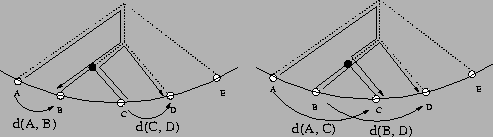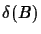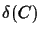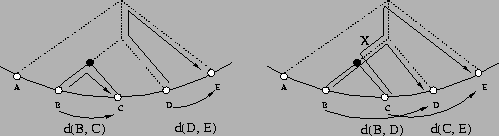Next: Algorithm Up: Tree Construction Algorithm Previous: Tree Construction Algorithm

## Idea of the algorithm

The basic idea of the tree construction algorithm is the following: write down the leaves in order of C. Starting from the leaves we want to find the internal nodes. Since we consider binary trees only, we know that at least two leaves are connected. When two leaves that are connected are swapped, the result a tree with the same topology. If we traverse the tree with the swapped leaves in the same circular order as before, we get the same path length. But when two leaves are swapped that are not connected (see Figure 3), some edges of the tree are traversed too often, which increases the score. So all we have to do is to swap each of the neighboring pair of leaves and to calculate the resulting total path length or score. If the score stays the same, we know that the two leaves are connected. If the score increases, the leaves are not connected. Since we use a TSP algorithm to find the order with the smallest score, the resulting score can never decrease. To save computation time we only recalculate the terms that actually change.Definition 4.1   Given is a tree T and a circular order C and leaves. Rename the leaves in a way s.t. the order of the leaves is in circular order C. We defineto be:Example 4.1:  Assume leaves B and C were connected (see figure 6). In the tree the distance d(A, B) plus d(C, D) is the same as the distance d(A, C) plus d(B, D) (when the leaves B and C are interchanged), because the tree topologies are identical. The difference between those two sumsis:is 2*x (if we try to swap the leaves (C, D)) (see Figure 7), because the two leaves are not connected. So with the functionwe can determine whether leaves si and si+1 are connected or not.
Note thatcorresponds exactly to the equation we used in the determination of the bound for the circular order. This means that the same error bound holds for the tree construction as for the circular order.Next: Algorithm Up: Tree Construction Algorithm Previous: Tree Construction Algorithm
Chantal Korostensky
1999-07-14